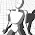Friday, November 11, 2011

Solving minesweeper using algebra

Language: English/Inglês What is in this post: Minesweeper linear systemMathematica solving the system
Have you ever wondered how to solve minesweeper in a mathematical way?
I have. It is pretty easy and straightforward. I'll show you a possible algorithm for this.
Consider the following  minesweeper puzzle:

I suppose you know the rules of minesweeper:  when a cell has a number 'x', it means that in the 8 cells around that cell, there is a total of 'x' mines. For example, around the '3' cell, there are 3 mines. The objective of the game is to pinpoint the location of all the mines. But for this, you need to make sure which squares don't have mines so you can click on them to get a number which will give you a new clue.

Ok, let's give names to the cells: I'll call $$x_1y_1$$ to the bottom left cell, $$x_1y_2$$ to the one above it, and $$x_2y_1$$ to the one at it's right, etc...

We need to express mathematically the information that is given to us. Our variables are gonna be the unknown cells around the number cells that are visible.Unknown cells around the number cells

The name of the each variable will be the name of the cell it represents. Each of the cell variables will have the value 1, if it has a mine, or 0 if it doesn't. It can't have the value 0.5 because a cell can't have half a mine, neither the value 2, because a cell has, at most, one mine.

Now it is easy to write what we see!

\begin{array}{lcl} \forall i,j : x_iy_j = 0 \lor x_iy_j = 1 \\ x_1y_1 + x_1y_2 = 1\\ x_1y_1 + x_1y_2 + x_1y_3 + x_2y_3 + x_3y_3 = 3\\ x_2y_3 + x_3y_3 + x_4y_3 = 1\\ x_3y_3 + x_4y_3 + x_5y_3 + x_5y_2 + x_5y_1 = 2\\ x_5y_2 + x_5y_1 = 2\\ \end{array}
Now I'll tell Wolfram Mathematica (a computer program) to solve this for me:

Simplify[Reduce[
{x1y1 + x1y2 == 1 &&
x1y1 + x1y2 + x1y3 + x2y3 + x3y3 == 3 &&
x2y3 + x3y3 + x4y3 == 1 &&
x3y3 + x4y3 + x5y3 + x5y2 + x5y1 == 2 &&
x5y2 + x5y1 == 2 &&
(x1y1 == 1 || x1y1 == 0) &&
(x1y2 == 1 || x1y2 == 0) &&
(x1y3 == 1 || x1y3 == 0) &&
(x2y3 == 1 || x2y3 == 0) &&
(x3y3 == 1 || x3y3 == 0) &&
(x4y3 == 1 || x4y3 == 0) &&
(x5y3 == 1 || x5y3 == 0) &&
(x5y2 == 1 || x5y2 == 0) &&
(x5y1 == 1 || x5y1 == 0)
}
, {x1y1, x1y2, x1y3, x2y3, x3y3, x4y3, x5y3, x5y2, x5y1}
]]

The output is:
x1y3 == 1 && x2y3 == 1 && x3y3 == 0 &&
x4y3 == 0 && x5y1 == 1 && x5y2 == 1 &&
x5y3 == 0 &&
((x1y1 == 0 && x1y2 == 1) || (x1y1 == 1 && x1y2 == 0))

This means that $$x_1y_3, x_2y_3, x_5y_1$$ and $$x_5y_2$$ cells have a mine while $$x_3y_3, x_4y_3$$ and $$x_5y_3$$ cells don't.
Whether or not the $$x_1y_1$$ and $$x_1y_2$$ cells have a mine is undetermined, but we know that if $$x_1y_1$$ has a mine, $$x_1y_2$$ doesn't and vice-versa.

What we do at this moment is to fill the board with the results we are 100% sure about. If we do so we get the following board:

As you can see, when we looked at the beginning board we couldn't immediately say where were the mines. It was not obvious. But using algebra gave us some help.
Now you just have to repeat this process until the board is solved.
Note that not every minesweeper puzzles have a determinable solution: which means sometimes every variable will be undetermined when you solve the system.
Also, in Microsoft minesweeper, there is a counter for how many mines are left not flagged at each moment. This could be helpful in near end game situations where you can't find out any cell value to be determined. However, i didn't include this data in the resolution I purposed for matters of simplicity. If you are curious about how to do it, post a comment.

1.Haha, it seems that I am not the only one to stumble upon this method. I came up with this method back in 2008 and there have been people that understood this method even earlier than us both. However, I have gone even further with my explanation and provided a working program that solves thousands of minesweeper grids. You can take a look if you like here: http://robertmassaioli.wordpress.com/2013/01/12/solving-minesweeper-with-matricies/

1.Nice! :). This is nothing new... it is a straight forward approach in operations research (Integer programming). I saw your blog where you say you want to make a parallel algorithm. This is probably doable in a disciplined way using a branch and bound (http://en.wikipedia.org/wiki/Branch_and_bound) algorithm where you simply don't care about the objective function (there is no objective function, only restrictions).

2.thanks for sharing valuable information

how to play minesweeper